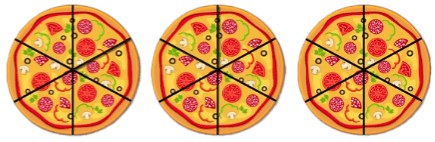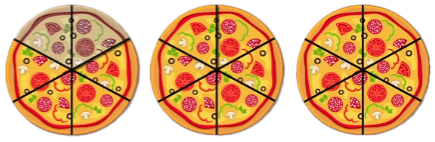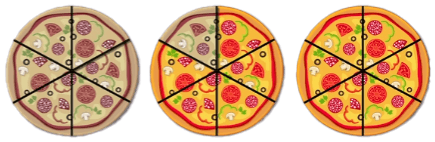# Proper and Improper Fractions and Mixed NumbersHi, and welcome to this review of fractions and mixed numbers!

Before we dive in, let’s review the basic parts of a fraction. Remember, a fraction simply represents a part of a whole. It has a numerator and a denominator, which tells us what the “part” is and what the “whole” is. Let’s look at the fraction $$\frac{3}{4}$$ as an example. We can see the 3 is our numerator and the 4 is our denominator. So the fraction $$\frac{3}{4}$$ is really saying 3 parts out of 4 parts total. It can also be helpful to visualize $$\frac{3}{4}$$ as simply

$$\frac{1}{4}+\frac{1}{4}+\frac{1}{4}$$

It’s very important to remember that a denominator of 4 does not represent the value of 4. A denominator of 4 represents the value of 1 that is divided up into 4 equal parts, or fourths. This type of fraction represents a value less than one whole. $$\frac{3}{4}$$ is not quite 1. If we had $$\frac{4}{4}$$ that would be equivalent to one, but we only have 3 out of 4 parts.

We see and use fractions that are less than one all the time in our daily lives, whether it’s for things like recipes or keeping track of time. Recipes often call for amounts such as “1/2 tsp salt,” and we often keep track of time in terms of quarter hours, like “a quarter past three” for 3:15. Though we observe this type of fraction very frequently in our daily lives, it is not the only type of fraction.

Consider the following scenario. You are ordering pizza for a big celebration. There will be a lot of hungry guests at this celebration, so you order 3 pizzas. Each pizza is cut into 6 slices. This means that each pizza has 6 equal parts, and as a fraction, 6 would be considered our “whole,” or our denominator.If your first guest eats 2 slices we would represent this as the fraction $$\frac{2}{6}$$. Two parts, out of 6 parts total.But what if that first guest was really hungry and grabbed 7 slices? Again, each pizza was cut into 6 equal slices, so 6 remains as our “whole,” or denominator. But this time our “part” is 7: $$\frac{7}{6}$$. In this scenario, our numerator is larger than our denominator.Fractions with a numerator larger than their denominator are referred to as improper fractions. Essentially, improper fractions equal a value that is more than one. One whole pizza would be represented by $$\frac{6}{6}$$, or “six sixths.” $$\frac{7}{6}$$ represents “seven sixths,” which is more than one pizza. This could be visualized as $$\frac{1}{6}+\frac{1}{6}+\frac{1}{6}+\frac{1}{6}+\frac{1}{6}+\frac{1}{6}+\frac{1}{6}=7$$. It can also be written in another form called a mixed number. An improper fraction and a mixed number will represent the same amount but simply be written in a different form.

For example, the improper fraction $$\frac{7}{6}$$ could also be written as the mixed number $$1\frac{1}{6}$$. Mixed numbers and improper fractions show the same amount, but as a mixed number, the “parts” are collected and consolidated into as many groups of 1 whole as possible. For example, $$\frac{4}{4}$$ would be grouped together as 1. $$\frac{7}{7}$$ would also be grouped together as 1. Any value where the numerator is equivalent to the denominator would be expressed simply as 1.

In our pizza example, the guest took 7 slices from a group of pizzas that were sliced into sixths. We said that this could be expressed as the improper fraction $$\frac{7}{6}$$, or visualized as $$\frac{1}{6}+\frac{1}{6}+\frac{1}{6}+\frac{1}{6}+\frac{1}{6}+\frac{1}{6}+\frac{1}{6}$$. As a mixed number, we would group 6 of these “sixths” in order to form $$1\frac{6}{6}$$, or 1 whole. By grouping $$\frac{6}{6}$$ together, we can see that $$\frac{1}{6}$$ is left over, on its own. We would write our mixed number as $$1\frac{1}{6}$$.

## Converting Improper Fractions to Mixed Numbers

Let’s try a few more examples. Let’s write the following improper fractions as mixed numbers: $$\frac{4}{3}$$ and $$\frac{3}{2}$$.

$$\frac{4}{3}$$ can be visualized as $$\frac{1}{3}+\frac{1}{3}+\frac{1}{3}+\frac{1}{3}$$. We know that $$\frac{3}{3}$$ is equal to 1, so let’s group 3 of these “thirds” together. We are now left with $$1\frac{1}{3}$$ as our mixed number.

$$\frac{3}{2}$$ can be visualized as $$\frac{1}{2}+\frac{1}{2}+\frac{1}{2}$$. We then know that $$\frac{2}{2}$$ makes one whole. And we’re left with $$\frac{1}{2}$$ left over. So $$\frac{3}{2}$$ as a mixed number is $$1\frac{1}{2}$$.

Let’s try one more example: $$\frac{7}{4}$$

$$\frac{7}{4}$$ is the same as $$\frac{1}{4}+\frac{1}{4}+\frac{1}{4}+\frac{1}{4}+\frac{1}{4}+\frac{1}{4}+\frac{1}{4}$$. Now we know that $$\frac{4}{4}$$ is grouped as one whole. So these $$\frac{4}{4}$$ pulled over, to equal 1. And we’re left with $$\frac{3}{4}$$. $$\frac{7}{4}$$ written as a mixed number is $$1\frac{3}{4}$$.

This process will take place in reverse in order to convert a mixed number to an improper fraction.

## Converting Mixed Numbers to Improper Fractions

For example, if we started with the mixed number $$1\frac{3}{4}$$ and we wanted to convert it to an equivalent improper fraction, we would take a look at the whole number, in this case, it is 1. This whole number is really representing our denominator that’s in the fraction. In this case, it’s 4, so the 1 is equal to $$\frac{4}{4}$$. When we combine these four fourths with the $$\frac{3}{4}$$, we end up with seven fourths total, or $$\frac{7}{4}$$.

That’s all there is to it! I hope that this video was helpful. Thanks for watching, and happy studying!

### What are examples of a proper fraction?

#### A

A proper fraction is a fraction that has no whole number part and its numerator is smaller than its denominator. Some examples of proper fractions are $$\frac{1}{4}$$,$$\frac{7}{9}$$,$$\frac{12}{13}$$,$$\frac{23}{25}$$, and $$\frac{17}{76}$$.

### What is a proper and an improper fraction?

#### A

A proper fraction is a fraction that has no whole number part and its numerator is smaller than its denominator. An improper fraction is a fraction that has a larger numerator than denominator and it represents a number greater than one.

Proper Fraction Examples: $$\frac{1}{2}$$, $$\frac{1}{6}$$, $$\frac{2}{5}$$, $$\frac{13}{14}$$, $$\frac{7}{11}$$

Improper Fraction Examples: $$\frac{16}{11}$$, $$\frac{12}{7}$$, $$\frac{6}{4}$$, $$\frac{3}{2}$$, $$\frac{8}{3}$$

### What is an improper fraction example?

#### A

$$\frac{17}{11}$$ is an example of an improper fraction because its numerator is greater than its denominator, which means it represents a value greater than one.

### What is a mixed number example?

#### A

A mixed number is a number that consists of a whole number part and a proper fractional part. $$4\frac{1}{3}$$ is an example of a mixed number because it has a whole number part $$(4)$$ and a proper fractional part $$(\frac{1}{3})$$.

### How do you turn an improper fraction into a mixed number?

#### A

To turn an improper fraction into a mixed number, figure out how many times the denominator can fit into the numerator and then how much of the numerator is left over. Then, the number of times the denominator fits into the numerator becomes the whole number part of the mixed number, and the number left over is the numerator of the fractional part over the original denominator.

Ex. Convert $$\frac{17}{4}$$ to a mixed number.
1) How many times can 4 fit into 17? 4 because $$4×4=16$$ – this becomes the whole number part
2) How much is left over in the numerator? 1 because $$17-16=1$$ – this becomes the numerator of the fractional part
3) $$\frac{17}{4}=4\frac{1}{4}$$

### How do you turn a mixed number into an improper fraction?

#### A

To turn a mixed number into an improper fraction, multiply the whole number part by the denominator and add the numerator. This becomes the new numerator over the original denominator.

Ex. Convert $$3\frac{5}{7}$$ to an improper fraction.

$$3\frac{5}{7}=\frac{3\times7+5}{7}=\frac{21+5}{7}=\frac{26}{7}$$

## Practice Questions

Question #1:

Which list of fractions contains amounts that are all more than one whole?

$$\frac{5}{3},\frac{7}{5},\text{ and }\frac{2}{2}$$
$$\frac{3}{5},\frac{7}{5},\text{ and }\frac{3}{2}$$
$$\frac{5}{3},\frac{7}{5},\text{ and }\frac{3}{2}$$
$$\frac{5}{5},\frac{7}{7},\text{ and }\frac{3}{3}$$

The correct answer is C: $$\frac{5}{3},\frac{7}{5},\text{ and }\frac{3}{2}$$.

When a fraction has a numerator larger than its denominator, the total value is more than one whole. Fractions like $$\frac{3}{3}, \frac{5}{5}, \text{ and }\frac{7}{7}$$ are all equal to one whole. Fractions with numerators less than their denominators represent values that are less than one whole. $$\frac{5}{3}, \frac{7}{5}, \text{ and }\frac{3}{2}$$ are all improper fractions representing total values larger than one whole.

Question #2:

Express the mixed number $$2\frac{1}{3}$$ as an improper fraction.

$$\frac{8}{3}$$
$$\frac{5}{3}$$
$$\frac{7}{3}$$
$$\frac{4}{3}$$

The correct answer is C: $$\frac{7}{3}$$.

$$2\frac{1}{3}$$ is equivalent to $$\frac{1}{3}+\frac{1}{3}+\frac{1}{3}+\frac{1}{3}+\frac{1}{3}+\frac{1}{3}+\frac{1}{3}$$. This represents seven groups of $$\frac{1}{3}$$, or as an improper fraction, $$\frac{7}{3}$$.

Question #3:

$$\frac{5}{3}$$ is equivalent to what mixed number?

$$1\frac{2}{3}$$
$$1\frac{3}{4}$$
$$2\frac{1}{3}$$
$$2\frac{2}{3}$$

The correct answer is A: $$1\frac{2}{3}$$.

$$\frac{5}{3}$$ can be thought of as $$\frac{1}{3}+\frac{1}{3}+\frac{1}{3}+\frac{1}{3}+\frac{1}{3}$$. We can combine three groups of $$\frac{1}{3}$$ in order to create $$\frac{3}{3}$$, or one whole. There are still two thirds left, so our mixed number is $$1\frac{2}{3}$$.

Question #4:

Mr. Jones orders sub sandwiches for his son’s basketball team. He orders eight subs and requests that they are each cut into three equal pieces. If his son eats two slices from one sub, what fraction of the total amount of food is left?$$\frac{7}{11}$$
$$\frac{11}{28}$$
$$\frac{11}{15}$$
$$\frac{11}{12}$$

The correct answer is D: $$\frac{11}{12}$$.

Eight subs, cut into three pieces each, creates twenty-four smaller sandwiches. Mr. Jones’ son eats two of these pieces, or $$\frac{2}{24}$$ from the total amount of food. This means that twenty-two pieces are left, or $$\frac{22}{24}$$. If the numerator and denominator are both divided by two, the simplified fraction becomes $$\frac{11}{12}$$. $$\frac{11}{12}$$ of the total amount of food is still remaining.

Question #5:

Kristina plans on drinking $$\frac{1}{4}$$ quart of water for every mile she runs. If she runs six miles, how much water will she drink?

$$1\frac{1}{4}$$ quarts of water

$$1\frac{1}{2}$$ quarts of water

$$2\frac{1}{2}$$ quarts of water

$$\frac{1}{2}$$ quarts of water

The correct answer is B: $$1\frac{1}{2}$$ quarts of water.

If Kristina drinks $$\frac{1}{4}$$ quart of water for every mile, she is essentially drinking $$\frac{1}{4}+\frac{1}{4}+\frac{1}{4}+\frac{1}{4}+\frac{1}{4}+\frac{1}{4}$$ quarts of water. The sum of this list of fractions is $$1\frac{2}{4}$$ or in simplest form, $$1\frac{1}{2}$$.